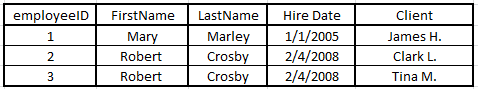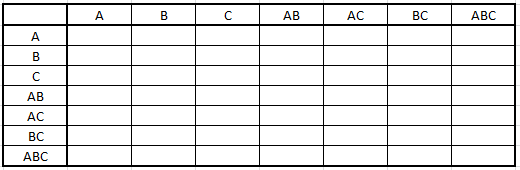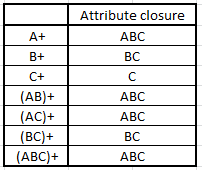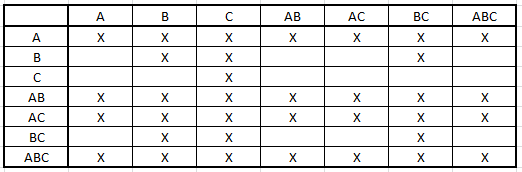# Intro to Database Systems : Schema Refinement - Functional Dependencies

Schema refinement is just a fancy term for saying polishing tables. It is the last step before considering physical design/tuning with typical workloads:

• 1) Requirement analysis : user needs
• 2) Conceptual design : high-level description, often using E/R diagrams
• 3) Logical design : from graphs to tables (relational schema)
• 4) Schema refinement : checking tables for redundancies and anomalies

Let’s see an example of redundancies and anomalies. Consider the following table where the client’s name is the primary key.The table is presenting information on employees (sales reps) and their clients.

If we want to insert data, we notice that:

• each row requires an entry in the client field
• we can’t insert data for newly hired sales reps until they’ve been assigned to one or more clients
• if sales reps are in a training process, even if they’ve been already hired, they can’t actually join the database because they need to have a delegated client… unless “dummy” clients are created.

If we want to update data, we notice that:

• the sales reps name is repeated for each client.
• what if, for a given client, we misspelled the name of the sales reps Crosby instead of Cosby… how can we edit that without affecting all the sales reps called Crosby?

If we want to delete data, what if Mary doesn’t have a client anymore because she’s taking a year off? We are forced to either

• create a dummy client
• incorrectly showing her with a client she no longer handled
• delete Mary’s record (even if however she’s still an employee)
• notice we can not have “null” as a client since primary field keys cannot store null.

When we have to treat with schema refinement we often notice that the main problem is redundancy. In order to identify schemas with such problems, we’ll introduce the notion of functional dependencies: a relationship that exists when one attribute uniquely determines another attribute. A functional dependency is simply a new type of constraint between two attributes.

Say that R is a relation with attributes X and Y, we say that there is a functional dependency X -> Y when Y is functionally dependent on X (where X is the determinant set and Y is the dependent attribute).

Let’s illustrate a scenario where the designer didn’t take in consideration dependencies between columns.

The following structure is considerably better:

• Course (courseID, courseName)

How do we pass from one to the other? That’s what schema refinement does through functional dependencies.

A unique way to represent a student is through his studID. Each student has his own address, hence we can say that studID determines address. We’ll write this in the following way:

In the previous example, we actually have the following FDs:

• studID - > studName, address
• courseID - > courseName
• studID, courseID - > grade

Let’s have a look at the properties of functional dependencies in the case where X, Y and Z are attributes belonging to a table R :

• transitivity: if we assume that X - > Y and Y - > Z, then it’s clear that X - > Z
• reflexivity: if Y is a subset of X, then X -> Y
• augmentation: if X - > Y, then for any Z we’ll have X, Z - > Y, Z
• union: if X - > Y and Y - > Z, then X - > Y, Z
• decomposition: if X -> Y, Z then X - > Y and X - > Z

The first 3 properties are called the Armstrong’s Axioms.

If F is a set of functional dependencies, F+ is the set of all FDs logically implied by F. Logically implied is just another way of saying obtained from the properties of functional dependencies ( the ones that we just enumerated). F+ is also called the closure of the set of functional dependencies. Is is the set of all dependencies logically implied by those present in F.

Let’s illustrate the usage of those properties with an example. If we have the following set of FDs, can we conclude that A - > H is logically implied?

• A - > B
• A - > C
• C, G - > H
• C, G - > I
• B - > H

Let’s see which properties are applicable to our case:

• We know that, by the transitivity property, if X -> Y and Y - > Z then we have X -> Z .
• In our case we have A - > B and B - > H.
• Hence, by transitivity, A - > H is logically implied.

Which other dependencies are part of the closure?

• CG -> HI by the union rule
• AG -> I by noticing that A -> C holds, and then AG -> CG by the augmentation rule and then AG -> I by transitivity.

Given a set of FDs, is there a faster way to compute if a dependency is logically implied?

Let’s see through an example how we can ask this question in multiple ways:

• Does F = {A - > B, B - > C, C D - > E} imply A - > E?
• Is A - > E in the closure F+ ?
• Is E in A+ ?

Before going on with a linear time algorithm, we notice that we’ve introduced a new notion, A+. We call A+ the attribute closure of A with respect to F and it will help us figure out if A - > E is logically implied.

• 1) Assume that we create a temporary attribute closure of A called TMP and that to begin, TMP = A (the input of the FDs that you want to verify)
• 2) Let’s consider the first given dependency of F, A - > B.
• 3) Is A in the TMP? Yes, since as stated previously TMP = A; we continue.
• 4) If we continue, we union B with the current TMP, A. What we obtain is the new TMP, AB (since A union B = AB).
• 5) We now consider the second given dependency, B - > C.
• 6) Is B in the TMP? Yes, since we now have AB in the TMP; we continue.
• 7) If we continue, we union C with the current TMP, AB. What we obtain is the new TMP, ABC (since AB union C = ABC).
• 8) We consider the 3rd given dependency, C D - > E.
• 9) Is CD in the TMP? No, since we only have ABC in the current TMP, hence we stop.
• 10) The attribute closure of A is then A+ = TMP = {A, B, C}

Now, to check if A - > E is in the closure F+, we can conclude that since E is NOT in A+, then A - > E is NOT in F+.

We can generalize this into an algorithm:

• 1) Consider the input of your FDs as the first element of your temporary attribute closure TMP.
• 2) Consider each dependency X - > Y of the given set of FDs
• 3) Is X part of TMP? If yes, continue to step 4. If no, continue to step 5.
• 4) Yes : Union TMP with Y.
• 5) No : Your attribute closure = TMP (your current temporary attribute closure from step 3).
• Conclusion : if an attribute is in your attribute closure, then it’s logically implied (it’s part of the closure of the set of functional dependencies).

Now that we know how to quickly verify if a dependency is logically implied… how do we find all the dependencies that are logically implied? Given a set of FDs F, how do we find its closure, F+ ?

Let’s go through an example again:

• Given F = { A - > B, B - > C}, compute F+

The algorithm is pretty simple:

1) Build an empty matrix with all possible combinations of attributes as rows and columns2) Compute the attribute closures of all attribute combinations3) Fill the matrix from step 1) by putting a check mark when a row member Y (from the table defined in step 1) is part of a member of the attribute closure Y+ (from the table defined in step 2) .Let’s look at some examples

• row member A: A, B, C, AB, AC, BC and ABC are all attributes of the closure of A+, ABC.
• row member BC. A is not a member of attribute closure (BC)+ : we don’t put a check mark because there is no A in (BC)+. However, we check B and C and BC.
• row member C. A is not a member of (C+): we don’t check it because C+ contains only C.

By having a check-mark at say the intersection of row A with column BC we mean that A - > BC is part of the closure F+. This is how we enumerate all the dependencies that are part of the closure F+.

Functional dependencies can also be used to find all the candidate keys. By definition, a candidate key is a set of columns that can be uniquely used to identify a database record without any irrelevant/unrelated/superfluous data. It is a reduction of the entire collection of attributes, hence a minimization.

Since we are talking about a minimal subset, we can start with the complete set of attributes and then, following functional dependencies, minimize the set until we reach the candidate keys (a set of attributes that can not be reduced). Let’s illustrate this once more by an example.

Say we have F = { A - > B, BC - > E and ED - > A}.

• 1) We know that the set of all attributes is ABCDE.
• 2) Can we reduce the set by using the first given FD? If we follow A - > B, we can remove B from the main set because B depends on A, and ABCD already contains A, hence no need of any dependent superfluous attribute. We obtain ACDE.
• 3) Can we reduce the set by using the second FD? If we follow BC - > E, we can remove E from the main set because E depends on BC, and ABCDE already contains BC. We obtain ABCD.
• 4) Can we reduce the set by using the third FD? If we follow ED - > A, we can remove A from the main set because A depends on ED, and ABCDE already contains ED. We obtain BCDE.
• 5) We now have a new set of attributes : ACDE, ABCD and BCDE. Let’s call them X.
• 6) Can we simplify any attribute from X by using dependency A - > B ? We can remove B from ABCD because ABCD already contains A, and B depends on A: we obtain ACD. Can we do the same for BCDE? No, because BCDE doesn’t contain A.
• 7) Can we simplify any attribute from X by using BC - > E ? We can remove E from BCDE because BCDE already contains BC and and E depends on BC: we obtain BCD. Can we do the same for ACDE ? No, because ACDE doesn’t contain BC,
• 8) Can we simplify any attribute from X by using ED - > A ? We can remove A from ACDE because ACDE already contains CD and A depends on CD: we obtain CDE.
• 9) We now have a new set of attributes : ACD, BCD and CDE. Let’s call them Y.
• 10) Can we simplify any attribute from Y by using A - > B ? BCD can not be simplified because it doesn’t contain A, and the rest of attributes from Y don’t contain B.
• 11) Can we simplify any attribute from Y by using BC - > E ? CDE can not be simplified because it doesn’t contain BC, and the rest of attributes from Y don’t contain E.
• 12) Can we simplify any attribute from Y by using ED - > A ? ACD can not be simplified because it doesn’t contain ED, and the rest of attributes from Y don’t contain ED.
• Conclusion: the functional dependencies from F can not be used to simplify the subsets from Y, hence they can not be more minimized. They are our candidate keys: ACD, BCD and CDE.

We notice that functional dependencies help us structuring our tables around unique attributes, avoiding superfluous information.